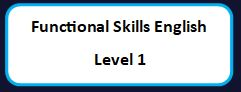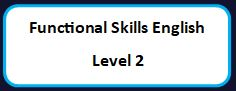# Maths Functional Skills

Our Maths Functional Skills platform is to proven equip learners with the skills and confidence to apply, combine and adapt their Mathematics knowledge to new situations in their life and work. It is concerned with developing and recognising the ability of learners to apply and transfer skills in ways that are appropriate to their situation.

You will develop your knowledge, understanding and confidence in all aspects of mathematical aspects such as

• Charts
• Graphs
• Positive and negative numbers
• Calculations
• Ratio
• Area and perimeters
• Fractions, decimals, and percentages
• Use simple formulae
• Measurements
• Recognise and use 2D and 3D objects
• Area, perimeter, and volume
• Use metric and imperial measures
• Collect and represent data
• Use and interpret statistical measures

On demand learning for any individual

Here is a few examples of our Maths Functional Skills lessons that are available to any individual on demand.

On the Maths Functional Skills platform, we are able to deliver

• Use of number and the number system,
• Use of common measures, shape and space,
• Handling information and data,
• Solving mathematical problems and decision making,

For Entry Level 1, Entry Level 2, Entry Level 2, Entry Level 3, Level 1 and Level 2.

You will also gain addition, subtraction, multiplication and division skills whilst being able to calculate using whole numbers, measurements and interpret numerical data.